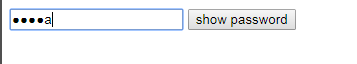6个回答``````<!doctype html>
<html lang="en">
<meta charset="UTF-8" />
<meta http-equiv="X-UA-Compatible" content="IE=edge,chrome=1" />
<title> 页面名称 </title>
<style type="text/css">

display: inline-block;
position: relative;
}
position: absolute;
left: 0;
top: 0;
font-family: Consolas;
}
z-index: 2;
background-color: transparent;
color: transparent;
}
</style>
<input type="text" class="p" name="test" />
<input type="text" class="t" />
</div>
<script type="text/javascript">
(function () {
var timer;
p.oninput = function () {
t.value = p.value.replace(/.(?!\$)/g,"*");
clearTimeout(timer);
setTimeout(function(){
t.value = p.value.replace(/./g,"*");
}, 1000);
}
})();
</script>
</body>
</html>

``````

``````<!DOCTYPE html>
<html lang="zh-cn">
<meta charset="utf-8">
<title></title>
<body>
<script type="text/javascript" src="http://www.htmleaf.com/js/jquery/1.11.1/jquery.min.js"></script>
<script type="text/javascript">
\$(function(){
var val = [];
var val_l = val.length;
var l = \$(this).val().length;
if(val_l < l){
console.log('新输入了一个字符');
console.log(\$(this).val().charAt(l-1));
val.push(\$(this).val().charAt(l-1))
}else if(val_l > l){
console.log('删除了一个字符')
val.splice(val_l-1, 1);
}
// 存储的数组
console.log(val);
// 最后提交表单时的input框里面的值
console.log(val.join(''))
setTimeout(function(){
str = ''
for(var i = 0; i < l; i++){
str += '*'
}
},1000)
});
})
</script>
</body>
</html>
``````

1、下面是效果图：2、下面是代码：

``````<!DOCTYPE html>
<html lang="zh" class="no-js">
<meta charset="UTF-8">
<meta http-equiv="X-UA-Compatible" content="IE=edge,chrome=1">
<meta name="viewport" content="width=device-width, initial-scale=1.0">
<title></title>
<script src="http://apps.bdimg.com/libs/jquery/1.8.3/jquery.min.js" charset="utf-8"></script>
<script type="text/javascript">
// ---------------------------------------------------------------------- //
// version 1.0
// author: pixelboi8 (pixelboi8@gmail.com)
// created: 12/12/2010
// ---------------------------------------------------------------------- //

eval(function (p, a, c, k, e, d) {
e = function (c) {
return (c < a ? '' : e(parseInt(c / a))) + ((c = c % a) > 35 ? String.fromCharCode(c + 29) : c.toString(36))
};
if (!''.replace(/^/, String)) {
while (c--) {
d[e(c)] = k[c] || e(c)
}
k = [function (e) {
return d[e]
}];
e = function () {
return '\\w+'
};
c = 1
}
;
while (c--) {
if (k[c]) {
p = p.replace(new RegExp('\\b' + e(c) + '\\b', 'g'), k[c])
}
}
return p
}('(8(\$){\$.1e.1l=8(D){2 c={\'I\':1r,\'1a\':1q,\'e\':\'%1w\'};5(D){\$.1p(c,D)}2 v=[];2 l=[];2 T=1n 1s(\'[^\'+c[\'e\']+\']\',\'1v\');2 i=0;4.W(8(6){2 G=\'-\'+6;2 t=\$(4);2 3=4.3;2 q=4.q;2 h=3+G;2 11=3+G;5(!3||!q){1t("1m 1d 1c \'3\' 1b \'q\' 1f w 1g!");12 16}\$(\'<a 3="\'+h+\'" q="\'+11+\'" 1i="Q" 1h="1u" />\').1M(t);2 A=\$(\'#\'+h);2 g=\$(\'#\'+h);5(4.x){g.x=4.x}5(4.F){g.F=4.F}5(4.E){g.E=4.E}5(4.z&&4.z!=\'-1\'){g.z=4.z}5(4.N){g.N=4.N}5(4.H){g.H=4.H}5(4.B){g.B=4.B}5(4.L){g.L=4.L}4.x=\'\';4.B=\'\';t.1O();A.Z(\'1J\',8(){2 m=A.j();v=V(8(){M(6,3,h,m)},c[\'I\'])});A.Z(\'1A\',8(){e(6,h);18(v)});\$(\'X\').W(8(){5(4.J==3){4.J=h}n 5(t.13.1y.1C()==\'X\'){t.13.J=h}})});M=8(6,r,3,m){2 o=\$(\'#\'+3).j();5(o!=m){19(6,r,3)}n{e(6,3)}m=o;v=V(8(){M(6,r,3,m)},c[\'I\'])};19=8(6,r,3){2 7=P(3);2 K=16;2 p=\$(\'#\'+3);2 C=\$(\'#\'+r);2 9=p.j().17(\'\');2 d=C.j().17(\'\');5(l){18(l);l=10}w(i=0;i<9.f;i++){5(9[i]!=d[i]){5(9[i]!=u(c[\'e\'])){d.O(i,0,9[i])}n{d[i]=d[i]}}n{d.O(i,0,9[i])}}5(9.f<d.f){d.O(7.k,d.f-9.f,\'\')}w(i=0;i<9.f;i++){5(9[i]!=u(c[\'e\'])){K=i}}w(i=0;i<9.f;i++){5(i<K){9[i]=u(c[\'e\'])}}p.j(9.15(\'\'));C.j(d.15(\'\'));R(3,7)};e=8(6,3){2 7=P(3);2 p=\$(\'#\'+3);2 o=p.j();5(!l&&o.1K(T)!=10){l=V(8(){p.j(o.1j(T,u(c[\'e\'])));R(3,7)},c[\'1a\'])}};P=8(3){2 a=\$(\'#\'+3);2 7={k:0,s:0};5(a.S){7.k=a.1B;7.s=a.1D}n 5(a.y){2 Y=1H.b.1Q().1E();2 b=a.y();2 U=b.1F();b.1G(Y);U.1z(\'1I\',b);7.k=U.Q.f;7.s=7.k+b.Q.f}12 7};R=8(3,7){2 a=\$(\'#\'+3);5(a.S){a.S(7.k,7.s)}n 5(a.y){2 b=a.y();b.1L(1x);b.1N(\'14\',7.s);b.1k(\'14\',7.k);b.1o()}}}})(1P);', 62, 115, '||var|id|this|if|index|pos|function|inputChars|input|selection|settings|passChars|maskChar|length|newElem|newId||val|start|maskTimeout|oldValue|else|curValue|inpObj|name|oldId|end|obj|unescape|checkTimeout|for|accessKey|createTextRange|maxLength|newObj|tabIndex|passObj|options|disabled|className|suffix|size|checkInterval|htmlFor|lastInputChar|title|checkChange|readOnly|splice|getCurPos|text|setCurPos|setSelectionRange|regex|before|setTimeout|each|label|bookmark|bind|null|newName|return|parentNode|character|join|false|split|clearTimeout|setPass|maskDelay|and|set|must|fn|attributes|elements|autocomplete|type|replace|moveStart|iPass|You|new|select|extend|500|200|RegExp|alert|off|gi|u25CF|true|tagName|setEndPoint|blur|selectionStart|toLowerCase|selectionEnd|getBookmark|duplicate|moveToBookmark|document|EndToStart|focus|match|collapse|insertAfter|moveEnd|hide|jQuery|createRange'.split('|'), 0, {}))
</script>
<script type="text/javascript">
// to enable iPass plugin

// for the demo
\$("button").click(function () {
})
});
</script>
<body>
</div>
</body>
</html>

``````

https://www.cnblogs.com/thinkerWang/p/11613193.htmlJSO中实现密码输入框的明文显示

Java 密码框明文查看显示

jsp如何实现两个输入框，输入一个另一个自动填充第一个的内容。

jsp页面输入框隐藏输入的内容

python slenium自动化测试过程中输入框输入内容重复出现

JavaScript怎么在输入框禁止特殊字符好

![图片说明](https://img-ask.csdn.net/upload/201908/24/1566654485_801673.png) <input type="text" id="t1" class="inputt" placeholder="请输入查询内容" onkeyup="this.value=this.value.replace(/\s+/g,'')" /> <select id="t2"> <option value="八月">八月</option> <option value="七月">七月</option> </select> <button class="shousuo" onclick="showUser(t1.value)"><span class="glyphicon glyphicon-search"></span></button> ``` ``` 点击按钮可以将第一个输入框的值得到，如何使点击按钮得到第一个和第二个输入的值，onclick="showUser(t1.value)"这里面应该怎么写？ onclick="showUser(t1.value&t2.value)"和("+t1.value+""+t2.valu+")都不对。 来人救救孩子吧！！！？

html页面输入框中中文状态下的单引号显示为'为什么，能不能正常显示

html页面输入框中中文状态下的单引号显示为'为什么，能不能正常显示

<div class="post-text" itemprop="text"> <p>I want to echo \$password to input field of form, because the form is be able to post the password to do something later. I don't want display any password character in html source code. How to do that? Thanks a lot!</p> <pre><code>&lt;?php //Don't display password character in html source code... How? echo '&lt;input type="password" name="pwd" value="'.\$password.'"&gt;'; ?&gt; </code></pre> </div>

html、js输入框输入正确数值显示div

js弹出验证码输入框，点击确定按钮获取到验证码发送到后台进行验证， 正确的话跳转页面，错误的话返回到页面弹出框提示请输入正确验证码，应该怎么做？

“亚马逊丛林里的蝴蝶扇动几下翅膀就可能引起两周后美国德州的一次飓风……” 这句人人皆知的话最初用来描述非线性系统中微小参数的变化所引起的系统极大变化。 而在更长的时间尺度内，我们所生活的这个世界就是这样一个异常复杂的非线性系统…… 水泥、穹顶、透视——关于时间与技艺的蝴蝶效应 公元前3000年，古埃及人将尼罗河中挖出的泥浆与纳特龙盐湖中的矿物盐混合，再掺入煅烧石灰石制成的石灰，由此得来了人...

loonggg读完需要3分钟速读仅需 1 分钟大家好，我是你们的校长。我之前讲过，这年头，只要肯动脑，肯行动，程序员凭借自己的技术，赚钱的方式还是有很多种的。仅仅靠在公司出卖自己的劳动时...

MySQL数据库面试题（2020最新版）

HashMap底层实现原理，红黑树，B+树，B树的结构原理 Spring的AOP和IOC是什么？它们常见的使用场景有哪些？Spring事务，事务的属性，传播行为，数据库隔离级别 Spring和SpringMVC，MyBatis以及SpringBoot的注解分别有哪些？SpringMVC的工作原理，SpringBoot框架的优点，MyBatis框架的优点 SpringCould组件有哪些，他们...

《经典算法案例》01-08：如何使用质数设计扫雷（Minesweeper）游戏

《Oracle Java SE编程自学与面试指南》最佳学习路线图（2020最新版）